# Trigonometry

The word ‘trigonometry’ means ‘triangle measurement’.It involves the ratios of the sides of right triangles and the angles. The main three ratios are called tangent, sin, and cosine.

Being one of the most important topics for the CAT exam, trigonometry requires the candidates to be well prepared with excessive practice to solve the related questions. There are some triangle questions which can be solved much faster if you can remember the trigonometric ratios involved, especially questions based on heights and distances. So, here is a detailed lesson on trigonometry for CAT topic along with various illustrations to help you understand the topic easily. There are three sides to a right-angled triangle which are named as follows depending on the angle.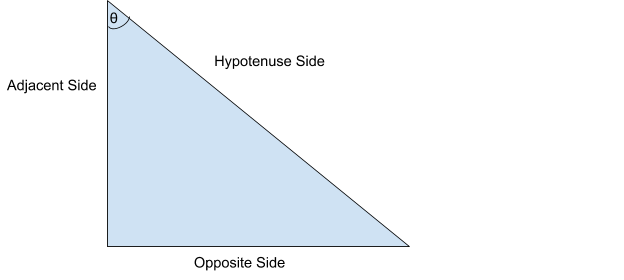• Opposite Side

The opposite side refers to the side opposite to the angle in question (θ). In this case, the opposite side is BC.

The Adjacent side is the side adjacent to angle θ. In this case, the adjacent side is AB.

• Hypotenuse Side

The hypotenuse side is always the longest side and the side opposite to the right angle.

In this case, the hypotenuse is AC.

Note: The adjacent and the opposite sides are marked depending on the angle θ. For the complementary angle of θ, the labels of the 2 sides are reversed.

## Trigonometric Functions

1. Sine of an angle:

The sine of an angle is the ratio of the opposite side to the hypotenuse side.

#### ∴ sin=opposite side/hypotenuse side

Sine is usually abbreviated as sin. Sin θ can be written as sin θ.

Example: Calculate the value of sin θ in the following triangle.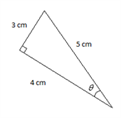Solution: sin θ = Opposite/Hypotenuse = 3/5.

2. Cosine of an angle: The cosine of an angle is the ratio of the adjacent side and hypotenuse side.

Cosine is usually abbreviated as cos. So, Cosine θ can be written as cos θ.

Example: Calculate the value of cos θ in the following triangle.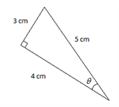3. Tangent of an angle:

#### The Tangent of an angle is the ratio of the opposite side and adjacent side.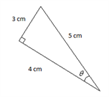In the given figure, Tanθ= opposite/adjacent = 3/4.

Note: There are three more trigonometric ratios which are the reciprocal of the Sin, Cos and tan angles respectively. They are:

1. Cosecant or Cosec θ = 1/ Sin θ.

2. Secant θ or Sec θ = 1/ Cos θ.

3. Cotangent or Cot θ = 1/ Tan θ.

## Special Triangles

There are two special triangles you need to know, 45-45-90 and 30-60-90 triangles. They are depicted in the figures below.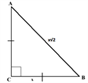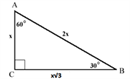The figures show how to find the side lengths of those types of triangles. Besides knowing how to find the length of any given side of the special triangles, you need to know their trigonometric ratio values (they are always the same, no matter the size of the triangle because the trigonometric ratios depend on the measure of the angle). The complete trigonometry chart is given below along with the values of individual trigonometric functions.

## Trigonometry Table: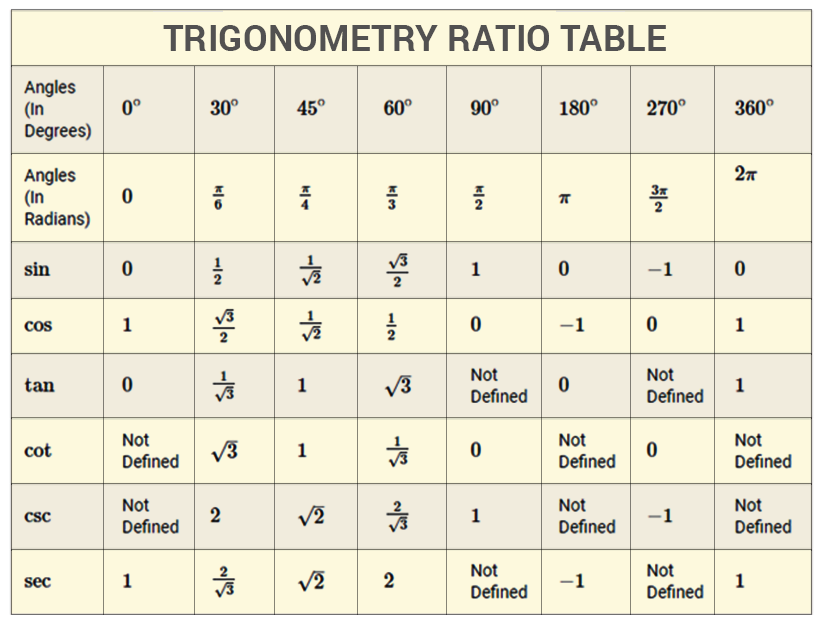Almost all questions can be solved by just using these ratios as log tables are not provided during the exam. Hence, you just need to know the sin, cos and tan values of these standard angles to solve all the related questions.

### ILLUSTRATIONS

Example 1:A ship is standing at some distance from a light house making an angle x with the top of the light house. There is a point D on the light house such that the angle of elevation of D is x/2. The distance of the point D from the ship is equal to the distance of D from the top of the light house. Find the ratio in which point D divides the lighthouse.

(a) 2:1

(b) 3:1

(c) 2: √5

(d) 3: √5

Solution: Option (a)

Method 1 Assume x=600 Then the angles will look as followsSince only ratio values are required, it can be assumed that CD=1. CDB is a 30-60-90 triangle. Hence, CB=3 & DB=2. Hence AD/DC=2:1. So, Option (a).

Method 2 Angle DBC= x/2, angle ABC = x, so angle ABD = x/2 As BD = AD, angle DAB = x/2 So, x/2+ x = 90 => x = 60 tan 60=AC/BC, tan 30=DC/BC => AC = 3PC. So the required ratio is 2:1.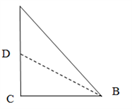Example 2: Anil looked up at the top of a lighthouse from his boat and found the angle of elevation to be 300. After sailing in a straight line 50 m towards the lighthouse, he found that the angle of elevation changed to 45o. Find the height of the lighthouse.

a) 25

b) 25√3

c) 25(√3-1)

d) 25(√3+1)

Solution: Option (d)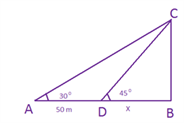Now since tan 45= 1, BC=DB= x Also, tan 30 =1/3= x/(50+x) Thus x(3 -1)= 50 or x= 25(3 +1) m. Example 3:An airplane flying at 3000 m above the ground passes vertically above another plane at an instant when the angle of elevation of the two planes from the same point on the ground are 600 and 450 respectively. The height of the lower plane from the ground is

a)1000√3 m

b) 1000/√3 m

c) 500 m

d) 1500(√3+1)

Solution:

Option (b)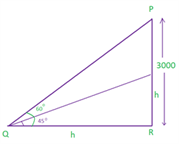Let the higher plane fly such that at point P the angle of elevation from point Q is 60o. Let the height of the plane flying at a lower level be “h” QR=h ( since tan 45o= 1) Tan 60o = 3000/h = 3 = > h= 10003.

With these illustrations, the aspirants can learn trigonometry for CAT in a cinch and with proper practice, the related question in the exam can be easily solved. It is also advised to know the latest CAT syllabus to properly get acquainted with all the important topics.

Stay tuned with BYJU’S and get lessons for all the important CAT exam topics along with the latest notifications and other details. Also, get various engaging video lesson to effectuate the CAT preparation.